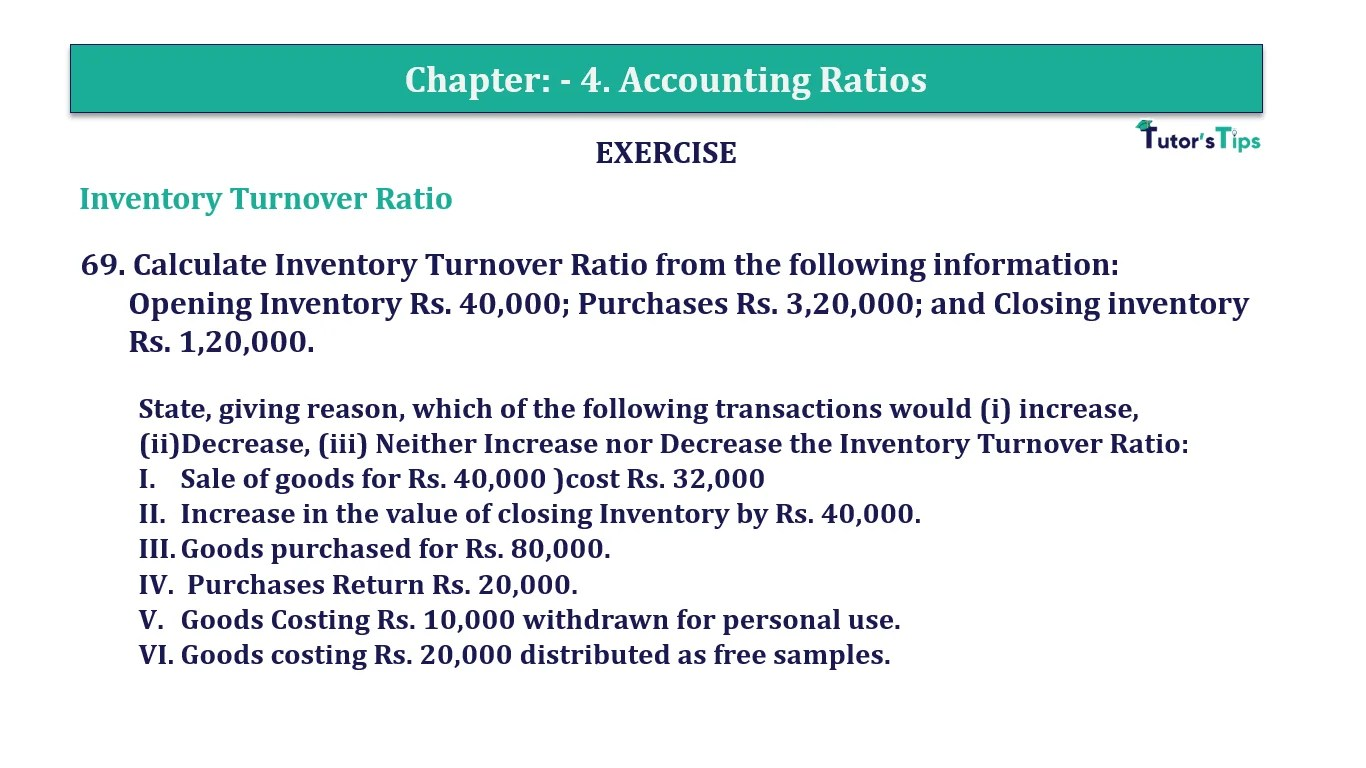# Question 69 Chapter 4 of +2-B – T.S. Grewal 12 ClassQuestion No. 69- Chapter No.4 - T.S. Grewal +2 Book Part B

Question 69 Chapter 4 of +2-B

Interest Coverage Ratio

69. Calculate Inventory Turnover Ratio from the following information:
Opening Inventory Rs. 40,000; Purchases Rs. 3,20,000; and Closing inventory Rs. 1,20,000.

State, giving reason, which of the following transactions would (i) increase, (ii)Decrease, (iii) Neither Increase nor Decrease the Inventory Turnover Ratio:

1. Sale of goods for Rs. 40,000 )cost Rs. 32,000
2. Increase in the value of closing Inventory by Rs. 40,000.
3. Goods purchased for Rs. 80,000.
4. Purchases Return Rs. 20,000.
5. Goods Costing Rs. 10,000 withdrawn for personal use.
6. Goods costing Rs. 20,000 distributed as free samples.

### The solution of Question 69 Chapter 4 of +2-B: –

 Cost of Goods of Goods Sold = Opening Stock +Purchases – Closing Stock = Rs. 40,000 + Rs. 3,20,000 – Rs. 1,20,000 = Rs. 2,40,000

 Average Inventory = Opening Inventory + Closing Inventory 2

 Average Inventory = Rs. 40,000 + Rs. 1,20,000 2 = Rs. 80,000

 Inventory Turnover Ratio = Cost of Goods of Goods Sold Average Stock

 Inventory Turnover Ratio = Rs. 2,40,000 Rs. 80,000 = 3 Times

 Transactions Impact on Inventory Turnover Ratio Reason (i) Sale of goods for Rs. 40,000 (cost Rs.32,000). Decrease The amount of closing stock is decreasing, increase in Cost of goods sold and decrease in Average Inventory. (ii) Increase in the value of closing Inventoryby Rs. 40,000.. Decrease There will be an increase in closing stock, the average stock is increased and a decrease in the cost of goods sold. (iii) Goods purchased for Rs. 80,000. Decrease The amount of average stock is increased but the amount of cost of goods sold is decreased. (iv) Purchases Return Rs. 20,000. Decrease The amount of average stock is decreased and increased in the amount of cost of goods sold. (v) Goods Costing Rs. 10,000 withdrawn for personal use. Increase The amount of average stock is decreased and increased in the amount of cost of goods sold. (vi) Goods costing Rs. 20,000 distributed asfree samples. Increase The amount of closing stock is decreased but the increase in Cost of goods sold.

Balance Sheet: Meaning, Format & Examples

Comment if you have any question.

Also, Check out the solved question of previous Chapters: –